: Randomness of the Digit : Curious Properties of Reiterated : The Digit Sum Process.

# 4. Loops of the Digit Sum Process.

By the Mathematica program in Example 10.4 we can calculate the loop for each non-negative integer n. The program is very simple. While generating the sequence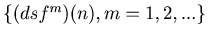, we store all the values of the previous terms. When we find that the same value of the sequence appears, we discover the loop. We have calculated loops for n = 1,2,...4000 by using this Mathematica function, and we have discovered the following 2 fixed points and 6 loops. In fact we are going to find later that these fixed points and loops are all the fixed points and loops of the digit sum process.

loop1A={1};

loop1B={3435};

loop2={421845123,16780890};

loop3={16777500,2520413,3418};

loop8={809265896,808491852,437755524,1657004,873583,
34381154,16780909,792488396};

loop11={791621579,776537851,19300779,776488094,422669176,
388384265,50381743,17604196,388337603,34424740,824599};

loop40={793312220,388244100,33554978,405027808,34381363,
16824237,17647707,3341086,16824184,33601606,140025,3388,
33554486,16830688,50424989,791621836,405114593,387427281,
35201810,16780376,18517643,17650825,17653671,1743552,
830081,33554462,53476,873607,18470986,421845378,34381644,
16824695,404294403,387421546,17651084,17650799,776537847,
20121452,3396,387467199};

loop97={1583236420,16827317,18470991,792441996,1163132183,
16823961,404291050,387424134,17601586,17697199,1163955211,
387473430,18424896,421022094,387421016,17647705,2520668,
16873662,17740759,389894501,808398820,454529386,404251154,
7025,826673,17694102,388290951,808398568,454579162,
388297455,421805001,16780606,17740730,2470915,388247419,
421799008,792442000,388244555,33564350,53244,3668,16870555,
17656792,389164017,405068190,404247746,1694771,389114489,
808395951,808401689,437799052,776491477,390761830,
405067961,388340728,51155506,59159,774847229,406668854,
33698038,421021659,387470537,19251281,404200841,16777992,
777358268,36074873,18471269,405068166,16920568,404294148,
404198735,405024914,387424389,421799034,775665066,1839961,
791664879,793358849,809222388,437752177,3297585,405027529,
388250548,50338186,33604269,387514116,17650826,17697202,
389114241,404198251,404201349,387421291,405021541,6770,
1693743,388290999};

Example 4.1.   Here we going to present the graphs of the loops. The graphs of loop2, loop3, loop8, loop11, loop40 and loop97 are Graphs 4.1, 4.2, 4.3, 4.4, 4.5 and 4.6.
Graph 4.1.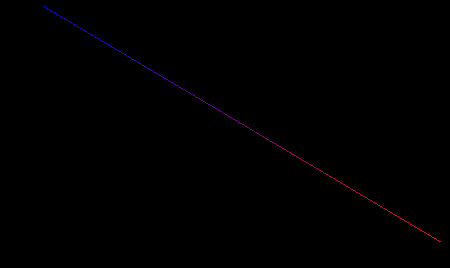Graph 4.2.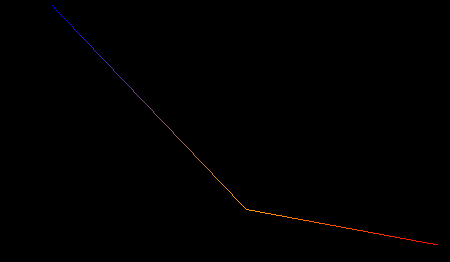Graph 4.3.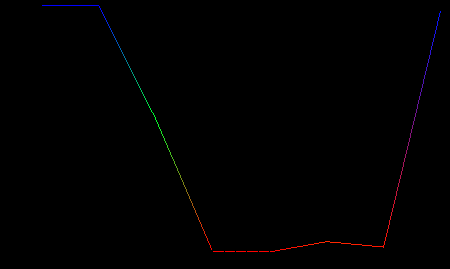Graph 4.4.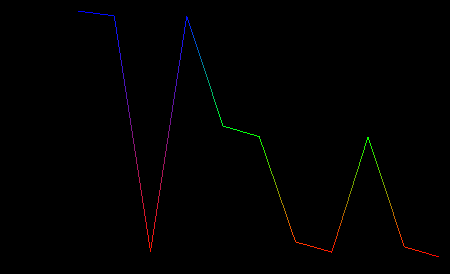Graph 4.5.Graph 4.6.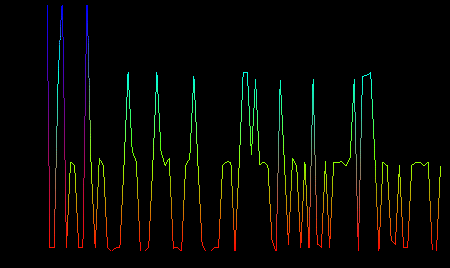Theorem 6.   For any non-negative integer n the sequenceeventually enters into one of the following 8 loops.
(1) loop1A = {1} with the length of 1.
(2) loop1B = {3435} with the length of 1.
(3) loop2 = {421845123,16780890} with the length of ２.
(4) loop3 = {16777500,2520413,3418} with the length of 3.
(5) loop8 = {809265896,..., 792488396} with the length of 8.
(6) loop11 = {791621579,... ,824599} with the length of 11.
(7) loop40 = {793312220,...,387467199} with the length of 40.
(8) loop97 = {1583236420,...,388290999} with the length of 97.
Remark. loop1A and loop1B are fixed points.

Proof.   By Theorem 4 and Theorem 5 we have only to prove for a non-negative number n such that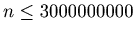.
We are going to use computer programs to prove this theorem, but it is very difficult to check the validity of computer programs. It is often the case that computer programs are only understandable to the person who made them. To ensure the correctness of the proof we are going to use two completely different computer programs. One is a Mathematica program, and the other is a C++ program. These two programs are made by two different persons who used two different algorithms.
The reason why we used two programs to ensure the correctness is that the calculation needs a lot of time and it is almost impossible to read all the output of the program with our own eyes.The first program is the Mathematica program in Example 10.5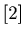The second program is the C++ program in Example 10.6 and Example 10.7. The program in Example 10.7 uses more than two computers at the same time. In other words this program use parallel computing. We used MPICH2 library for our program.．
Here we present the speed comparison of these two C++ programs.

$time ./a.out 1 1000000 > out real 0m19.910s user 0m15.668s sys 0m3.697s$ time mpiexec -n 2 ./a.out 1 1000000

real	0m5.065s
user	0m0.097s
sys	0m0.039s

For example, if we calculate for n =1,...,1000000, then the amount of time used by these two programs are 19.91 versus 5.065. Approximately 4 : 1.

Remark 4.1.   6 loops in Theorem6 are registered at the data-base of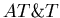Labs-Research as,,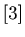,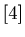,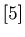and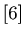.

Example 4.2.   By the program in Example 10.7 we can get the data for each number, and if we apply the program in Example 10.8, we can find which loop each number eventually enter into and how many steps each number needs to enter into it.

Here we present the number of n such thatenters into each fixed point or loop.
For example, if you see Graph 4.7, then you can find out that the number of n such thatenters into loop3 is 12436682.

Graph 4.7.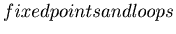the number of n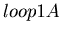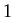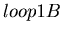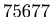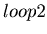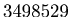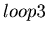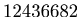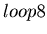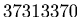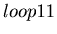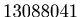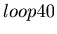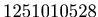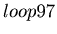Example 4.3.   By the program in Example 10.9 we have calculate the number of steps that each number needs to enter into a loop. One of the number less than 3000000000 with the longest total stopping time is 1022355577 with 124 steps.

Graph 4.8.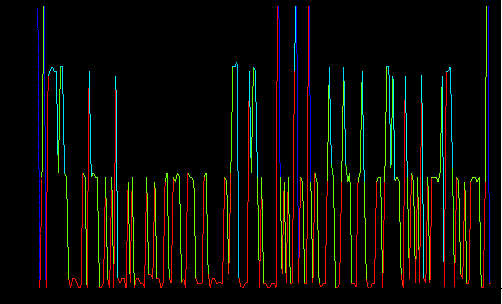: Randomness of the Digit : Curious Properties of Reiterated : The Digit Sum Process.